Follow

Basic Math - Adding, Subtracting, Multiplying, Dividing

Overview

In this article we will go over how to Add, Subtract, Multiply and Divide in the Calculation Field. We will be showing you basic functionality. In later articles, we will go over more advanced calculations.

In this example I have a form where I am adding together the price of apple and the price of bread. I have two numeric fields Price of Apple and Price of Bread.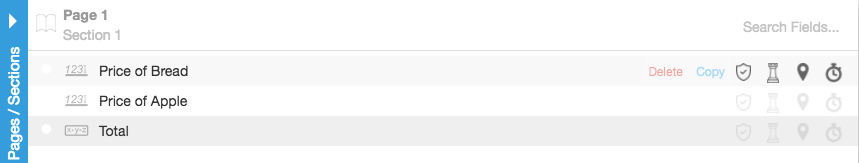``````Price of Apple - \$Apple_1\$

As you can see, in the Calculation field Total I have added the two fields together making sure to include the “\$” symbol before and after each fieldkey.

``\$Apple_1\$ + \$Bread_1\$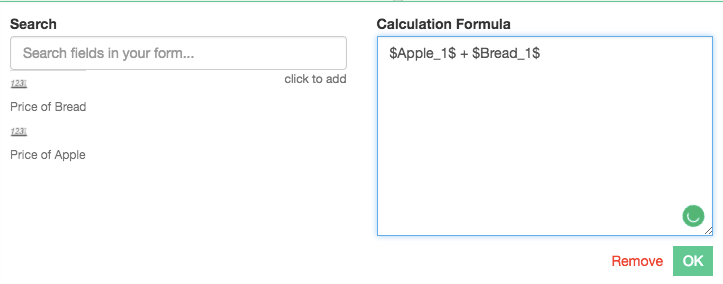``

Note: Because I am calculating a price, I will need to make sure to allow for 2 decimal places in the builder for each of my numeric fields and also for my calculation field. See picture belowAfter saving and publishing the form, I will now go into the app and fill out those two fields. My Total field will add those two fields together and show the totalSubtracting

We will be using the same example as the Adding section, but we will be modifying it a little bit. Instead of adding those two fields together we will now subtract them. I will now be subtracting Price of Bread from Price of Apple.``````Price of Bread - \$Bread_1\$
subtracted from
Price of Apple - \$Apple_1\$``````

In the Calculation field Total, I have subtracted the two fields from each other

``\$Apple_1\$ - \$Bread_1\$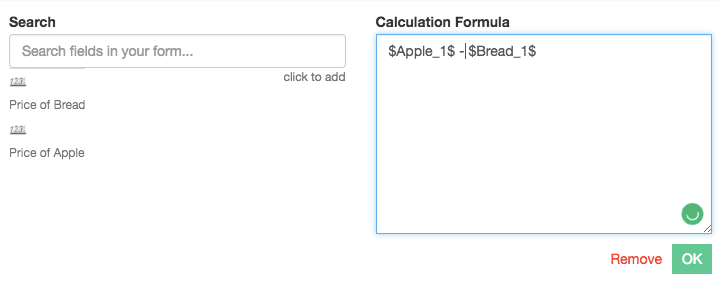``

Note: Because I am calculating a price, I will need to make sure to allow for 2 decimal places in the builder for each of my numeric fields and also for my calculation field. See picture belowAfter saving and publishing the form, I will now go into the app and fill out those two fields. My Total field will subtract those fields from each otherMultiplying

We will be using the same example as the Adding section, but we will be adding onto it a little bit to demonstrate how to multiply using the calculation field.

On the form, I have added two numeric fields and named them Quantity and placed them in between Price of Apple and Price of Bread. We will be multiplying Price of Apple by Quantity, also Price of Bread by Quantity. I have also put two new Calculation fields on the form Total Price of Apples and Total Price of Bread. We will be calculating each of the totals separately, though you could use the same calculation field to total all of it at once, for demonstration purposes I’ll keep it simple.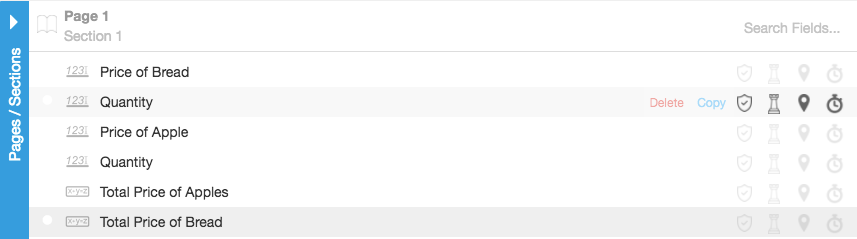Just for review, these are the fields and their associated fieldkeys.

Example: Apples

``````Price of Apple - \$Apple_1\$
multiplied by
Quantity - \$Qty_Apple\$``````

The calculation inside of your Total Price of Apples will look like this

``\$Apple_1\$ * \$Qty_Apple\$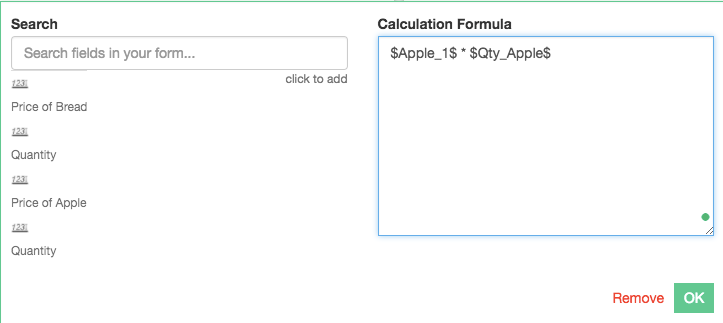``

This is what it looks like in the app after filling it outIt will be the same thing for the bread; As follows

``````Price of Bread - \$Bread_1\$
multiplied by

The calculation inside of your Total Price of Bread will look like this

``\$Bread_1\$ * \$Qty_Bread\$``

This is what it looks like in the app after filling it outDividing

Hopefully, if you’ve followed along with the article up to this point you can probably guess how to do this next part. It really is as easy as you might think.

In this next example, we will be using some multiplication, addition, and division to get an average price. Although this section is focusing on showing you how to divide in the calculation field, you will also see some basic algebra and how that might be accomplished in your own calculations.

Also, in this example, I am using many calculation fields to demonstrate my point. This example could have been completed, and in many cases would be preferable to use just a single calculation field to get your desired result.

In this example, I have Price of Bread 1 and Price of Bread 2. Each of those fields has their own Quantity fields below each of them. We will be taking the price of bread, and multiplying it by the quantity to get the Total Price of Bread. Then we will add those two totals together to get the Total Price. Then will get the average price by taking the Total Price and dividing it by 2. The number of Total Price of Bread fields that we have. This will give us the Average Price of Bread. Below is a screenshot of all of the fields and their names.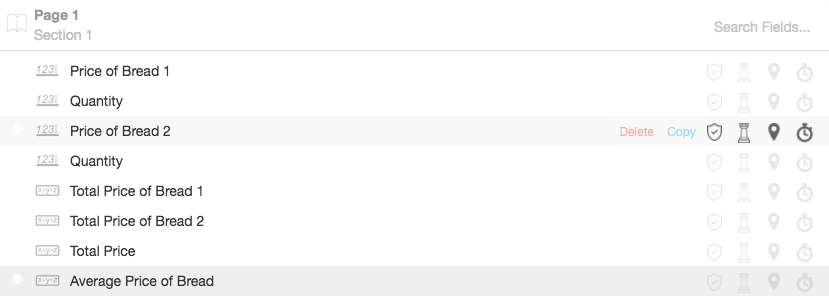Below are the calculation field names, their fieldkeys in (parenthesis) and their contained calculations follow the = equal sign.

``````Total Price of Bread 1 (total_bread_1) = \$price_bread_1\$ * \$qty_bread_1\$